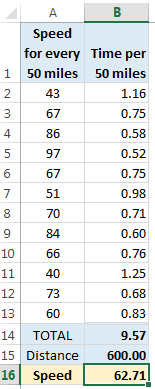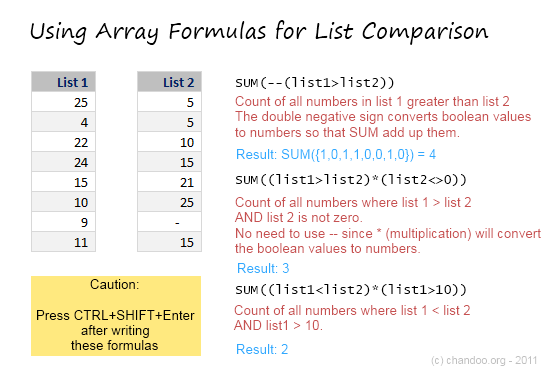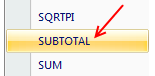# All articles with 'sum()' Tag

## Weighted Average in Excel [Formulas]

Published on Sep 19, 2019 in Learn ExcelLearn how to calculate weighted averages in excel using formulas. In this article we will learn what a weighted average is and how to Excel’s SUMPRODUCT formula to calculate weighted average / weighted mean.

What is weighted average?

Wikipedia defines weighted average as, “The weighted mean is similar to an arithmetic mean …, where instead of each of the data points contributing equally to the final average, some data points contribute more than others.”

Calculating weighted averages in excel is not straight forward as there is no built-in formula. But we can use SUMPRODUCT formula to easily calculate them. Read on to find out how.

Continue »

## What is the average speed of this road trip? [Solution & Discussion]

Published on Aug 18, 2014 in Excel Howtos, Formula ForensicsLast week, we had an interesting homework problem – What is the average speed of this road trip?

We received more than 150 answers. But to my surprise, 57 of them are wrong. So today, lets learn how to calculate the average speed correct way.

Continue »

## CP007: aweSUM() – Overview of SUM functions in Excel

Published on May 1, 2014 in Chandoo.org Podcast Sessions, Learn ExcelIn the 7th session of Chandoo.org podcast, lets make you aweSUM().

Imagine for a second that Excel cannot add up numbers. And no it cant subtract them either. What would that look like?

A glorified Notepad. That’s right. Excel’s ability to add up numbers, along with features like formulas, charts, pivot tables & BHATTEXT() are what make it such a lovely software. May be not the BHATTEXT(), but we all agree that Excel is so versatile and useful because it can add up numbers (and perform other calculations) with ease.

But how well do you know the SUM formulas of Excel?

In this podcast, you will learn,

• Special personal fruit announcement 😛
• + operator
• Status bar & total rows in tables
• Auto Sum feature
• SUM() function
• SUMIFS function
• Special cases of SUMIFS function
• SUBTOTAL & AGGREGATE functions
• Other summing functions – SUMPRODUCT etc.
Continue »

## Formula Forensics 022. Sum the Odd Numbers between 1 and 100

Published on May 24, 2012 in Formula Forensics, Huis, Posts by HuiHow can we Add Up the Odd numbers between 1 and 100 ?

Continue »

## Formula Forensics. No 007 – Sumproduct

Published on Dec 21, 2011 in Formula Forensics, Huis, Learn Excel, Posts by HuiOne of the most asked questions within the posts and Forums at Chandoo.org is, How Does Sumproduct work ?

Today in Formula Forensics we take at look at just that with a few worked examples.

Continue »

## Formula Forensics No. 002 – Joyces Question

Published on Nov 7, 2011 in Excel Howtos, Formula Forensics, Huis, Posts by HuiFormula Forensics # 002 – Joyces Question

This is the second post of a new regular weekly series at Chanoo.org, Formula Forensics, where we will examine how a formula works from the inside out with a worked example to help you understand its formation.

Last week we looked at Taruns problem and analysed a formula to solve his problem

This week we look at Joyce’s Problem…

Continue »

## Sum of Values Between 2 Dates [Excel Formulas]

Published on Sep 27, 2011 in Excel Howtos, Learn ExcelLets just say, you run a nice little orange shop called, “Joe’s Awesome Oranges“. And being an Excel buff, you record the daily sales in to a workbook, in this format. After recording the sales for a couple of months, you got a refreshing idea, why not analyze the sales between any given 2 dates? […]

Continue »

## Accumulated Depreciation using Mixed References

Published on Jul 19, 2011 in Excel Howtos, Financial ModelingLast time we had discussed the use of SumProduct() to ease your life for calculation of consolidated revenues and depreciation. This time we would be using the sum function! Yes you heard it right – The Sum function.

But we would use the Sum function with a small trick! We would use it to calculate running cumulative sum! And believe me, you would need this function so many times – to calculate accumulated depreciation, cumulative debt, Profits to Retained Earnings and almost all the accounts that would consolidate into the balance sheet.

Continue »

## Comparing Lists of Values in Excel using Array Formulas

Published on Jun 14, 2011 in Excel Howtos, Learn ExcelLast week, we had a home work on Calculating Donation Summaries using Excel Formulas. This is a good case where array formulas can help us. So today, we will learn how we can use Array Formulas to compare lists of values and calculate summaries. Towards the end of this post, you can see a video that explains the solution to Donation Summary Calculation problem.

Continue »

## Amount Donated vs. Pledged [Excel Formula Homework]

Published on Jun 10, 2011 in Excel ChallengesWe have some home work folks! Today, lets test your Excel formula skills by giving some data related to a fund.

The problem:
You manage a fund for a non-profit. You have donors who pledge certain amount at the start of the year. As you go thru the year, the donors donate money to your fund. At the end of the year, you have a table like above. And you need to summarize the fund’s performance by calculating various statistics.

Go

Continue »

## Scheduling Variable Feed Sources

Published on Nov 18, 2010 in Excel Howtos, Posts by HuiIn many industries, bulk commodities are received or delivered in batches or parcels of various quantities and with various properties.
This post will look at one option for scheduling these commodities within Excel.

Continue »

## How to Look up Based on Multiple Conditions

Published on Nov 2, 2010 in Excel Howtos, Learn ExcelSituationNot always we want to lookup values based on one search parameter. For eg. Imagine you have data like below and you want to find how much sales Joseph made in January 2007 in North region for product “Fast car”? Read more to find how to solve this.

Continue »

## What is Excel SUBTOTAL formula and 5 reasons why you should use it

Published on Feb 9, 2010 in Learn ExcelToday we will learn Excel SUBTOTAL formula and 5 beautiful reasons why you should give it a try.

SUBTOTAL formula is used to find out subtotal of a given range of cells. You give SUBTOTAL two things – (1) a range of data (2) type of subtotal. In return, SUBTOTAL will give you the subtotal for that data. Unlike SUM, AVERAGE, COUNT etc. which do one thing and only one thing, SUBTOTAL is versatile. You can use it to sum up, average, count a bunch of cells.

Continue »

## 29 Excel Formula Tips for all Occasions [and proof that PHD readers truly rock]

Published on Aug 24, 2009 in Excel Howtos, Featured, Learn Excel

It is no exaggeration that knowing excel formulas can give you a career boost. From someone starting at the long list of numbers, you can suddenly become a data god who can lookup, manipulate and analyze any spreadsheet.

So when our little excel blog hit the 5000 RSS Subscriber milestone, I celebrated the occasion by asking you to share an excel formula through twitter or comments with rest of us. And boy, what an excellent list of formula tips you have shared with us all.
Here is the complete list of entries for the twitter formula contest.

Continue »

## Sumif with multiple conditions [quick tip]

Published on Jul 22, 2009 in Excel HowtosHere is a little formula trick if you need to sum a range of cells based on multiple conditions.

Assuming you have the starfleet, captain and flight data, you can use the good old sum() in an array formula to conditionally sum values meeting multiple criteria. Read on to learn this quick tip.

Continue »

### Get FREE Excel & Power-BI Newsletter

One email per week with Excel and Power BI goodness. Join 100,000+ others and get it free.